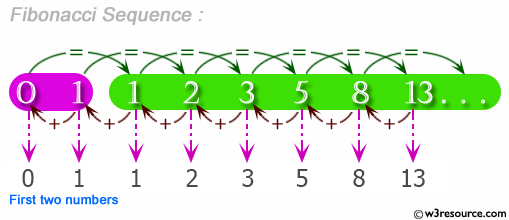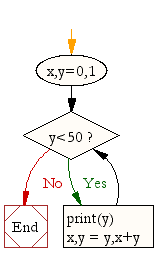﻿ Python Exercise: Fibonacci series between 0 to 50 - w3resource# Python Exercise: Fibonacci series between 0 to 50

## Python Conditional: Exercise-9 with Solution

Write a Python program to get the Fibonacci series between 0 to 50.

Note : The Fibonacci Sequence is the series of numbers :
0, 1, 1, 2, 3, 5, 8, 13, 21, ....
Every next number is found by adding up the two numbers before it.

Pictorial Presentation:Sample Solution:

Python Code:

``````x,y=0,1

while y<50:
print(y)
x,y = y,x+y
```
```

Sample Output:

```1
1
2
3
5
8
13
21
34
```

Flowchart:## Visualize Python code execution:

The following tool visualize what the computer is doing step-by-step as it executes the said program:

Python Code Editor :

Have another way to solve this solution? Contribute your code (and comments) through Disqus.

What is the difficulty level of this exercise?

Test your Python skills with w3resource's quiz

﻿

## Python: Tips of the Day

Returns a list with n elements removed from the beginning

Example:

```def tips_take(itr, n = 1):
return itr[:n]
print(tips_take([1, 2, 3], 5))
print(tips_take([1, 2, 3], 0))
```

Output:

```[1, 2, 3]
[]
```